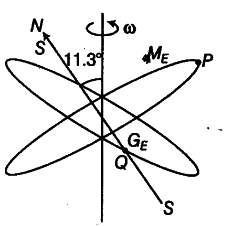# Obtain the declination and dip angles at P and Q

Consider the plane S formed by the dipole axis and the axis of the earth. Let P be point on the magnetic equator in S. Let Q be the point of intersection of the geographical and magnetic equators. Obtain the declination and dip angles at P and Q.

P is in the plane of S, needle is in North, so the declination is zero.P is also on the magnetic equator, so the angle of dip is zero, because the value of angle of dip at equator is zero. Q is also on the magnetic equator, so the angle of dip is zero.
As, the earth is tilted on its axis by 11.3°, thus the declination at Q is 11.3°.